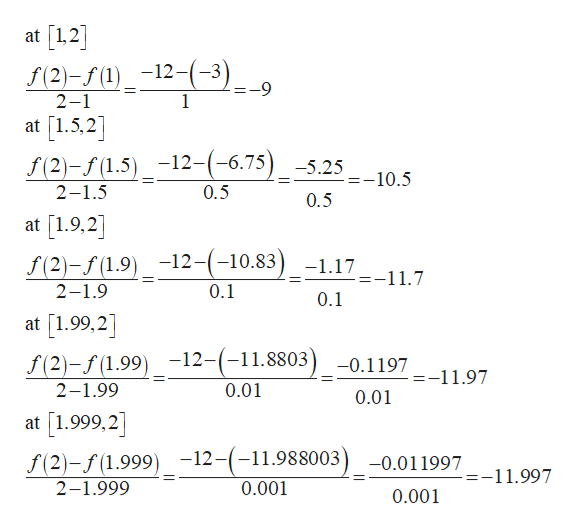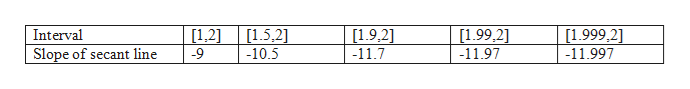For the function​f(x)=-3x^2make a table of slopes of secant lines and make a conjecture about the slope of the tangent line at x=2.Interval [1,2]

Question

For the function

​f(x)=-3x^2

make a table of slopes of secant lines and make a conjecture about the slope of the tangent line at x=2.

Interval [1,2]

Step 1

It is known that the slope of a secant line between points (a, f(a)) and (b, f(b)) is given by:

Step 2

Now, evaluate slopes of secant line at different intervals between the provided interval [1,2]:help_outlineImage Transcriptioncloseat 1,2 -2(9) at 1.5,2 f(2)-(L.5)-12-(-6.75) f(2)-f(1)12-(-3 2-1 1 -5.25 -10.5 2-1.5 0.5 0.5 at [1.9,2 f(2)-f(1.9) 12-(-10.83) -1.17_11.7 2-1.9 0.1 0.1 at 199,2] f (2)-f(1.99)-12-(-11.8803) -0.1197 --11.97 2-1.99 0.01 0.01 [1.999,2 at f(2)-f(1.999)-12-(- 2-1.999 -11.988003)-0.011997 =-11.997 0.001 0.001 fullscreen
Step 3

So, the table of slopes of seca...help_outlineImage TranscriptioncloseInterval [1.9,2] -11.7 [1.999,2] -11.997 [1,2] -9 [1.5,21 -10.5 [1.99,2] -11.97 Slope of secant line fullscreen

Want to see the full answer?

See Solution

Want to see this answer and more?

Our solutions are written by experts, many with advanced degrees, and available 24/7

See Solution
Tagged in

Calculus